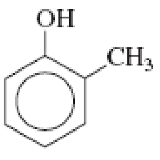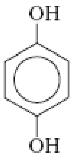Chapter 3, Problem 3.75EP### Organic And Biological Chemistry

7th Edition
STOKER + 1 other
ISBN: 9781305081079

#### Solutions

Chapter
Section### Organic And Biological Chemistry

7th Edition
STOKER + 1 other
ISBN: 9781305081079
Textbook Problem

# Name the following phenols.(a)

Interpretation Introduction

Interpretation:

The given phenol has to be named.

Concept Introduction:

When one hydrogen atoms are replaced by a hydroxyl group in benzene is known as phenol.  If the other hydrogen atoms present in phenol are replaced by other atoms or groups are known as phenol derivatives.

Phenol derivative with one substituent:

IUPAC system of naming phenol derivative considers phenol to be a part of main name.  The numbering is given in a way that the substituent gets the least numbering possible.

Ortho- means disubstitution in 1,2

Meta- means disubstitution in 1,3

Para- means disubstitution in 1,4

In all the above derivatives of phenol, the first position is occupied by hydroxyl group.

Phenol derivatives with two or more substituents:

More than two groups are present in the benzene ring means, their positions are numbered.  The numbering is always done in a way that the carbon atom bearing substituent gets the lowest numbering possible.  If there is a choice of numbering system, then the group that comes alphabetically first is given the lowest number.

Explanation

Given structure is,

The above structure contains a hydroxyl group attached to a benzene ring.  Therefore, in the name phenol has to be added as suffix.  Numbering has to be done considering the hydroxyl group as number 1...

(b)

Interpretation Introduction

Interpretation:

The given phenol has to be named.

Concept Introduction:

When one hydrogen atoms are replaced by a hydroxyl group in benzene is known as phenol.  If the other hydrogen atoms present in phenol are replaced by other atoms or groups are known as phenol derivatives.

Phenol derivative with one substituent:

IUPAC system of naming phenol derivative considers phenol to be a part of main name.  The numbering is given in a way that the substituent gets the least numbering possible.

Ortho- means disubstitution in 1,2

Meta- means disubstitution in 1,3

Para- means disubstitution in 1,4

In all the above derivatives of phenol, the first position is occupied by hydroxyl group.

Phenol derivatives with two or more substituents:

More than two groups are present in the benzene ring means, their positions are numbered.  The numbering is always done in a way that the carbon atom bearing substituent gets the lowest numbering possible.  If there is a choice of numbering system, then the group that comes alphabetically first is given the lowest number.

(c)

Interpretation Introduction

Interpretation:

The given phenol has to be named.

Concept Introduction:

When one hydrogen atoms are replaced by a hydroxyl group in benzene is known as phenol.  If the other hydrogen atoms present in phenol are replaced by other atoms or groups are known as phenol derivatives.

Phenol derivative with one substituent:

IUPAC system of naming phenol derivative considers phenol to be a part of main name.  The numbering is given in a way that the substituent gets the least numbering possible.

Ortho- means disubstitution in 1,2

Meta- means disubstitution in 1,3

Para- means disubstitution in 1,4

In all the above derivatives of phenol, the first position is occupied by hydroxyl group.

Phenol derivatives with two or more substituents:

More than two groups are present in the benzene ring means, their positions are numbered.  The numbering is always done in a way that the carbon atom bearing substituent gets the lowest numbering possible.  If there is a choice of numbering system, then the group that comes alphabetically first is given the lowest number.

(d)

Interpretation Introduction

Interpretation:

The given phenol has to be named.

Concept Introduction:

When one hydrogen atoms are replaced by a hydroxyl group in benzene is known as phenol.  If the other hydrogen atoms present in phenol are replaced by other atoms or groups are known as phenol derivatives.

Phenol derivative with one substituent:

IUPAC system of naming phenol derivative considers phenol to be a part of main name.  The numbering is given in a way that the substituent gets the least numbering possible.

Ortho- means disubstitution in 1,2

Meta- means disubstitution in 1,3

Para- means disubstitution in 1,4

In all the above derivatives of phenol, the first position is occupied by hydroxyl group.

Phenol derivatives with two or more substituents:

More than two groups are present in the benzene ring means, their positions are numbered.  The numbering is always done in a way that the carbon atom bearing substituent gets the lowest numbering possible.  If there is a choice of numbering system, then the group that comes alphabetically first is given the lowest number.

### Still sussing out bartleby?

Check out a sample textbook solution.

See a sample solution

#### The Solution to Your Study Problems

Bartleby provides explanations to thousands of textbook problems written by our experts, many with advanced degrees!

Get Started